# Output Power

As you have probably noticed, my knowledge base articles are free of advertising. Instead of distracting you with annoying ads, I kindly request your donation. If you find the contents of this page to be useful, please consider making a donation by clicking the Donate button below.Output Power

Perhaps the most frequently asked question regarding the LM3886 chip amplifier is, “how much power can I get out of the LM3886?” My aim with this section is to answer that question as well as provide some insight into the limitations imposed by the LM3886 and how these limitations impact the output power.

Section outline

Topology Number of LM3886es Recommended Supply Voltage Output Power
8 Ω 4 Ω
Single 1 ±28 V 38 W 68 W
Parallel 2 ±35 V 60 W 100 W
Bridge (BTL) 2 Not recommended.
Bridge-Parallel (BPA) 4 ±28 V 150 W 270 W
Bridge-Parallel (BPA) 6 ±35 V 200 W 400 W
Recommendations assume the amplifier should be capable of driving a 4 Ω speaker load.

The quick estimate

The power dissipated in a resistor by a sinusoidal voltage can be calculated from the RMS value of the applied voltage: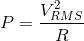For amplifier calculations, it is often more convenient to use the peak voltage, in which case the dissipated power can be calculated as:This equation allows the output power of any amplifier to be estimated as long as its supply voltage is known. Assuming no voltage drop across the output devices of the amplifier and a symmetrical power supply (i.e. ±VCC), the peak output voltage becomes the supply voltage and the output power can be estimated as:For slightly higher precision on the estimate, I suggest including the collector-emitter saturation voltage of the output stage (labeled clipping voltage in the LM3886 data sheet):The clipping voltage is found in Figures 14 and 15 in the LM3886 data sheet; reproduced below.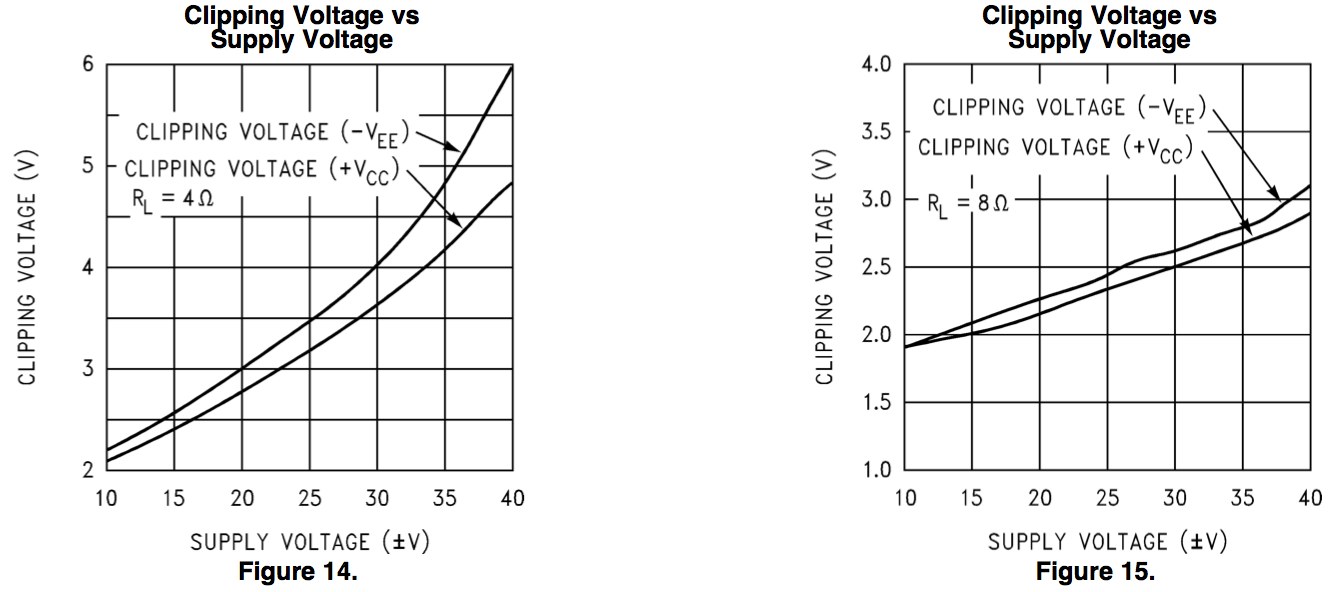Note that the clipping voltage varies as function of the supply voltage. Also note that the clipping voltage is likely measured at DC, hence any output power calculation using this parameter assumes that the LM3886 reproduces a sine wave cleanly all the way to the DC clipping voltage. Thus, the power numbers estimated here are a touch optimistic. For example, when using a ±28 V power supply and driving an 8 Ω load, the LM3886 has a clipping voltage of 2.6 V as illustrated below.It should therefore be able to produce an output power ofor slightly higher than the 38 W (THD < 0.1 %) specified in the data sheet.

The output power of the LM3886 is limited by three parameters: The maximum supply voltage, the maximum output current, and the power dissipated in the LM3886 itself.

As shown in the spec table in the data sheet, the maximum output current the LM3886 is guaranteed to be able to provide is 7 A (peak).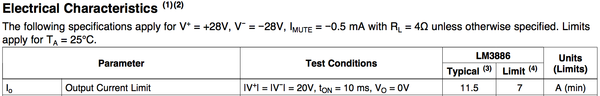Following Ohm’s Law, this means the peak output voltage the LM3886 can produce into a 4 Ω load is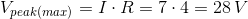As result the maximum recommended supply voltage with a 4 Ω load is ±28 V, resulting in 68 W of output power at below 0.1 % THD.

In addition to the output current limit, the power dissipated in the LM3886 itself imposes another limit on the supply voltage. The power dissipated in the LM3886 versus supply voltage and output power is shown in the figure below.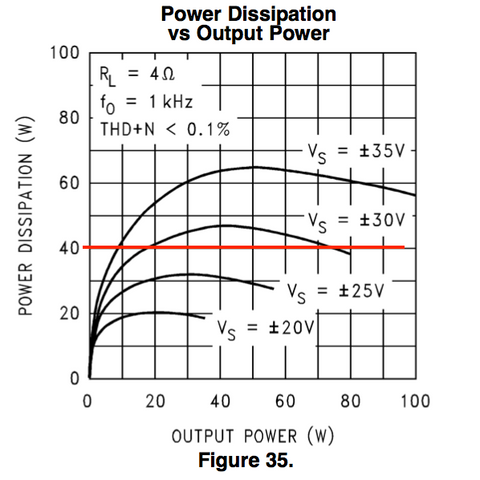The BPA200 application note (TI Application Note AN-1192) recommends designing for a maximum dissipated power of 40 W, indicated by the red line on the graph. As seen in the above figure, this limits the supply voltage to ±28 V (±27.6 V to be exact), hence my recommendation of a ±28 V maximum supply voltage when using a 4 Ω load. For those interested in how this graph came about and how to calculate the power dissipated in a Class AB output stage, please see the Thermal Design section.

Following the same math, the supply voltage with an 8 Ω load should be ±56 V but, as shown below, this is beyond the maximum supply voltage allowed by the LM3886.Thus, with an 8 Ω load, the supply voltage could at first glance be the maximum specified ±42 V. There are two issues with this approach: Firstly, operating an IC at the specified absolute maximum supply voltage leaves no margin for supply voltage variation, thus risks permanently damaging the IC. Secondly, operating the LM3886 at such high supply voltage dissipates well over 40 W in the LM3886 itself as shown below.As mentioned previously, TI recommends a maximum power dissipation in the LM3886 of 40 W. This recommendation is supported by the pulse power limitation of the LM3886 as shown in the graph below.Note that the pulse power limit decreases sharply at rising voltage. Thus, to stay within the limits of the LM3886 output devices, the supply voltage should be limited to ±35 to ±36 V even with an 8 Ω load. Using a supply voltage of ±35 V the LM3886 provides 60 W into 8 Ω (THD < 0.1 %).

Parallel, bridge, or both?

For those who desire higher output power than the 38 W (8 Ω) and 68 W (4 Ω) the LM3886 can provide often consider connecting multiple LM3886es in parallel, bridge (BTL), or a combination of bridge and parallel. Of these, the parallel option provides the most reliable performance.

Parallel

When two amplifiers are connected in parallel they share the load current. Another way to put this is that each amp half “sees” twice the load impedance. This means two LM3886es in parallel driving a 4 Ω load follows the “8 Ω math” above. Hence, two LM3886es in parallel can drive a 4 Ω load when operated on a ±35 V power supply. In this configuration, the total output power is 100 W (4 Ω) and 60 W (8 Ω). Up to 40 W is dissipated in each LM3886 in this configuration and they must be properly heat sunk to be able to deliver the full output power.

Do note that when connecting two LM3886es in parallel, ballast resistors must be used to ensure that the two LM3886es share the load current evenly. Also note that the input voltage offset difference between the two LM3886es causes a standing current to flow through the ballast resistors. This standing current must be minimized to avoid excessive THD even at moderate output power levels. To accomplish this, the input offset of each LM3886 must be minimized, preferably using a DC servo thereby increasing the complexity of the circuit considerably.

Bridge

Connecting two LM3886es in a bridge (BTL) configuration increases the output power by doubling the output voltage swing. Unfortunately, this means that in a BTL configuration each amp half “sees” half the load impedance. Thus, driving an 8 Ω load limits the power supply voltage to ±28 V to avoid exceeding the 7 A output current limit of the LM3886 (V = I · R; V = 7 · 8/2 = 28 V). To drive a 4 Ω load the supply voltage will need to be lowered to ±14 V for a total output swing of 28 V, peak (not including the clipping voltage). The result is an amplifier that provides performance similar to a single LM3886 at more than twice the build cost of a single LM3886 amplifier. I therefore do not recommend bridge/BTL operation with the LM3886 if both 4 Ω and 8 Ω loads are to be supported by the amplifier.

Bridge-Parallel

A final option in the quest for higher output power is to build two parallel LM3886 amplifiers and connect those in a bridge configuration resulting in a Bridge-Parallel Amplifier (hence the BPA200 name of the National Semiconductor/TI application note linked to earlier). In the original BPA200 application note four LM3886es were used in a BPA configuration to build a “200 W” amplifier. This will work well on a ±28 V power supply and provide approximately 150 W (8 Ω), 270 W (4 Ω).

For even higher output power, the supply voltage can be increased to ±35 V. However, to stay below the 7 A output current limit of each LM3886, three LM3886es should be used in each amp half. Thus, the total amplifier will contain six (6!) LM3886es per mono block. Recall,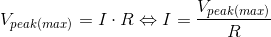Also recall that in a bridged amp, the amp delivers the full VCC-VEE swing across the load (so 70 V peak swing across the load from a ±35 V supply). Hence for a BPA amp to drive a 4 Ω load to clipping on a ±35 V supply, it must be able to deliver 70/4 = 17.5 A of output current. This requires 17.5/7 = 2.5 LM3886es to accomplish, thus each amp half must be built using three LM3886es in parallel with accompanying ballast resistors and DC servos. Needless to say, this is not a “beginner level” circuit by any stretch of imagination. If implemented correctly and provided with adequate heat sinking, such an amplifier should be able to deliver 200 W into 8 Ω and 400 W into 4 Ω.

Implications for the Modulus product line

As the Modulus-86 uses one LM3886 it shares the same limitations as the fundamental LM3886 circuit regarding output power. I therefore recommend using a ±28 V power supply with Modulus-86 to allow support for both 4 Ω and 8 Ω speakers. The Modulus-86 is not recommended for bridging.

If the Modulus-86 does not provide enough power for you, the best option in the current Modulus line-up is the Modulus-286. When operated on ±35 V supply rails it provides 60 W (8 Ω), 100 W (4 Ω). Two Modulus-286 modules may be bridged and operated on a ±28 V supply for 150 W (8 Ω), 270 W (4 Ω) of output power.

For even higher output power, the Modulus-686 provides 220 W (8 Ω), and 380 W (4 Ω) when operated on a ±36 V supply, and is capable of driving a 2 Ω load.

Section 5: Thermal Design